4132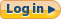| Help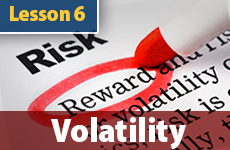# Lesson 6 - Volatility

by Uncle Bob Williams

Volatility & Standard Deviation

Volatility is the most important factor to understand when trading Options because it has a key role in determining the price of the Options. There are 2 kinds of Volatility, Historical Volatility and Implied Volatility.

The Volatility allows us to calculate the Standard Deviation. The Standard Deviation is the gauge to understand and to evaluate price changes.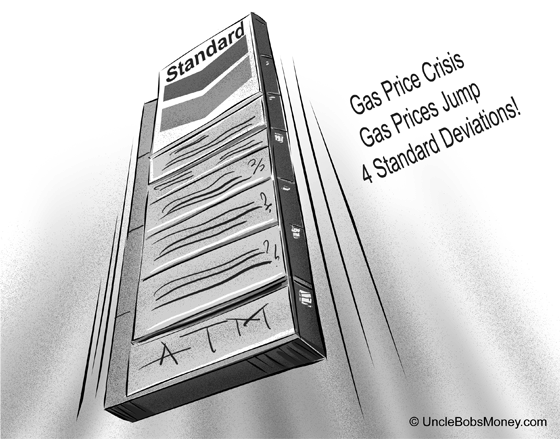For Example:

If the price of gasoline suddenly jumped up \$1.00 per gallon more than it was last week when we bought gas, we would be shocked. When we purchase gasoline for our car every week, we normally expect a price change of say \$0.05 or \$0.10 per week, so if we saw that the price went up \$1.00 we would know that something dramatic happened to gas prices.

Using Statistics we can take a more methodical approach to understand how dramatic the price change was and how likely it is to happen. If we look at the history of the gas prices, we could calculate the Weekly Standard Deviation. Let's say, just for example purposes, that One Standard Deviation for a week is \$0.10. Statistically, we know that 1 Standard Deviation equals 68% of the activity or approximately 2/3rds of the time. So most of the time, the price of gas shouldn't go up or down by more than \$0.10 every week.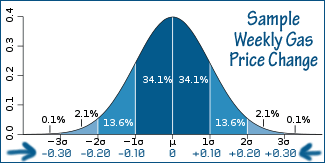2 Standard Deviations represents 96% of the activity, so most of the time, the price of gas shouldn't go up or down by more than \$0.20 in a week. To say it a little more clearly, we expect that 2/3rds of the time that the price change will be less than \$0.10 (which is the 1 Standard Deviation Range), we also expect that around 1/4th of the time, the price change may be as high as \$0.10 to \$0.20 (which is the range of 2 Standard Deviations). So, if the price changed \$0.20, it would be unusual, but still normal.

3 Standard Deviations represents 99.7% of the activity, so we would expect that the price of gas shouldn't go up or down by more than \$0.30 in a week. The chance of the price going up or down by more than \$0.30 in a week is very small. We expect that 2/3rds of the time that the price change will be less than \$0.10 (which is the 1 Standard Deviation Range), we also expect that around 1/4th of the time, the price change may be as high as \$0.10 to \$0.20 (which is the range of 2 Standard Deviations), and we only expect the price change to be between \$0.20 to \$0.30 around 4% of the time or only once or twice a year.

4 Standard Deviations represents 99.99% of the activity. This means that the price change would be between \$0.30 and \$0.40 around 0.3% of the time - which is approximately only once every 5 to 6 years.

So, if we knew that the Standard Deviation for the change in weekly gasoline prices is \$0.10, and we arrive at the gas station to find that the price had suddenly went up \$1.00, we would immediately start checking the news to find out what happened. We would be statistically confident that there was a gas embargo or some other serious event that caused the price of gas to jump up so high so suddenly.

The Volatility and the resulting Standard Deviation is very important for Options trading because the Volatility is the gauge to understand and evaluate how much risk we have when we trade Options.

Let's refer back to our earlier example of Insurance, where we said that Options are like insurance. An Insurance Company will look at the claims history and general accident statistics to figure out how much risk they are taking if they issue us an insurance policy and approximately how much it will cost them to pay us if we ever have a claim against them. They can then figure out how much to charge us, so they will be able to make a profit.

With Options trading, we don't have a team of PhDs in Statistics to help us evaluate the risk for each trade, but we don't need them because we have both the Historical and Implied Volatility numbers which tell us the amount of statistical risk that each Options trade has.

- - - - -

Historical Volatility

Historical Volatility describes how much price movement a stock or Index had during a specific period. If we look a chart of the price for our fictitious company Acme stock over the last 30 days, it is possible to calculate what the Historical Volatility for Acme stock was during that period. The Historical Volatility will tell us the Standard Deviation of how much the price of Acme stocked changed each day. The Standard Deviation tells us statistically how much the typical daily price movements were, and it sounds more complicated than it is.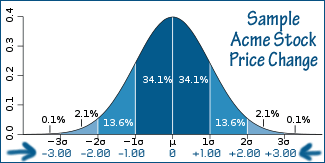For example:

The current price of Acme Stock is \$50 per share.

The Standard Deviation for the price of Acme Stock over the last 30 days is 1.

This means that 68.2689% of the time during the last month, the price change of Acme stock fluctuated in a range that was not more than \$1.00. The price change could have been zero, or only a few cents. But it was not more than \$1.00. In other words, about 2 days out of every 3 days, the price change was not greater than \$1.00.

2 Standard Deviations represents 95.4499736% of all the activity. This means that the 2 Standard Deviation range represents a little more than a quarter of the time (28%) where the price change was between \$1.00 to \$2.00.

3 Standard Deviations represents 99.7300204% of the activity. This means that the 3 Standard Deviation range represents only 4% of the time. We would therefore expect to see a price change between \$2.00 and \$3.00, one time out of every 25 days.

4 Standard Deviations represents 99.993666% of the activity. This means that the 4 Standard Deviation range represents only 0.3% of the time. We would therefore expect to see a price change between \$3.00 and \$4.00, one time out of every 333 days. Which would be a rare event.

5 Standard Deviations represents 99.9999426697% of the activity. This means that the 5 Standard Deviation range represents only 0.0000573303% of the time. We would therefore expect to see a price change between \$4.00 and \$5.00, one time out of every 15,787 days (once every 43 and 1/4 years IF the trading happened every day of the week). Which would be extremely, extremely rare.

6 Standard Deviations represents 99.9999998027% of the activity. This means that the 6 Standard Deviation range represents only 0.0000001973% of the time. We would therefore expect to see a price change between \$5.00 and \$6.00, one time out of every 1,744,278 days (once every 4,778 and 3/4 years IF the trading happened every day of the week). Which would be an astronomically rare event.

We use the Historical Volatility as the yard stick to measure the fear level of the market when we compare it to the Implied Volatility, and to measure if the current Options pricing is Expensive or Cheap when compared to the Theoretical Option price using Implied Volatility.

NOTE: The Stock Market does not follow a perfect Normal Distribution (Standard Deviation) Curve. There are times when the Market has a 'crash' and the price movement is greater than 6 Standard Deviations, even though we said above that such an event would not likely happen except for once every 4,778+ years.

So, are we fooling ourselves? Is it realistic to use a Normal Distribution (Standard Deviation) Curve when the rules get broken?

We find it appropriate to use the Normal Distribution (Standard Deviation) Curve as long as we realize that the ends of the curve, when discussing Market movements, are not flat like a Normal Distribution (Standard Deviation) Curve. When we discuss market movements, we have to keep in mind that there are outside forces and that a 'crash' can occur.

Instead of throwing out the Normal Distribution (Standard Deviation) Curve, instead we embrace it when we plan our 'normal' trading strategies, and we can now also use it as a tool to plan for and hedge against a 'crash': and we are able to get great pricing on our 'hedge' positions exactly because of our understanding of the Normal Curve. Since the Market will price Options that are far out on the curve according to the statistical probabilities, instead of the actual History which includes market crashes, it can often be to our advantage and we are able to buy positions that act as Insurance against a big crash at a cost that is less than the actual risk.

- - - - -

Implied (Intrinsic) Volatility

Implied Volatility describes how much demand there is for specific Options. We should think of Implied Volatility as price, instead of a calculation of a series of price numbers, because the Implied Volatility is calculated based on the demand for Options and not on a series of numbers that happened in the past. Based on the demand for specific Options, we can establish the Implied Volatility and use that to calculate the Theoretical Option price based on the volume of the demand.

Implied Volatility is the Fear Gauge

Implied Volatility can be thought of as the Fear Gauge for Options trading.

When people get worried that the Market might make a big move (usually down), they will start buying more Options as Insurance to protect their stock positions against loss if the market has a big drop. Remember we said originally that you can only buy an Option if someone is willing to sell one, so when the demand for Options goes up, the Options pricing goes up as well. The increase in the Options demand is reflected as a rise in the Implied Volatility.

Implied Volatility then can be used as a gauge to measure market fear. If the Implied Volatility goes up or stays high, it's an indication that people are worried that the Market might have a downturn. Likewise, when the Market is moving up, people are less nervous and the Implied Volatility will generally go down.

Some of the Strategies we trade at Uncle Bob's Money are dependent on the Implied Volatility both in terms of the range (high/low) and whether the trend is going up or down. The Uncle Bob's Money Trading Checklist automatically takes these Implied Volatility movements into account and it will both show when it is OK to trade specific strategies and when you should exit those strategies. A Calendar Strategy for example can become a lot more profitable when the Implied Volatility goes UP, and it can become a loss if the Implied Volatility goes down too much.

Expensive or Cheap?

We said that when demand goes up, the price goes up. But in a normal market, we can use the Implied Volatility to understand if the Options we want to trade are expensive or cheap.

If the Implied Volatility is the same as the Historical Volatility, then the Market is pricing the Options about the same as the Historical price movements.

If the Implied Volatility is LOWER than the Historical Volatility, then the Market expects the price of this Stock / Index to be very stable in the near future. We would say in this case that the Option price is "cheap" because the Market is pricing the Options lower than the Historical price movements of this Stock / Index.

Likewise if the Implied Volatility is HIGHER than the Historical Volatility, then the Market expects the price of this Stock / Index to be more turbulent in the near future. We would call these Options "expensive" because the Market is pricing the Options higher than the Historical price movements of this Stock / Index.

NOTE: For the sake of consistency, we will consider the general Implied Volatility for a specific stock / Index as the Implied Volatility for the "AT THE MONEY" CALL position for the current month expiration.

When we calculate the Standard Deviation at Uncle Bob's Money, that is always the value we use. We use this number as a GUIDE, and never as an absolute reference. The mathematically inclined can dive deep into Options Math - but for our purpose there is no need to get into the gritty math details. If for example, we want to make a Double Diagonal trade, where our Short positions are approximately 1 Standard Deviation away from the current Market price, any small Implied Volatility difference won't have an effect on the trades we select. Once we know approximately which Short Strikes to select, we then will evaluate each specific trade to determine which one we want to enter.

Let's use our fictitious Acme company for an example:

The Historical Volatility for Acme stock shares is 20%.
The current Implied Volatility for the Acme "At The Money" CALL is also 20%.

In this case, the Historical Volatility and the Implied Volatility are the same or very close, so we know that the price of the Options reflects the approximate price movements of the stock/Index.

If the Implied Volatility was high, it means that that the price of the Options would be high because there is excessive demand for those Options: IE: People are worried.

Market Changing Event:

Acme might have an earnings announcement, or there might be a natural disaster that affects their supply chain, or they may lose a key person, or there might be a general Market downturn or event that affects all stocks. When one of these events happens, the Market will be worried about price drops and the demand for Options will go up, along with the price for those Options.

 Have a Question? Comment? Suggestion? Ask us.